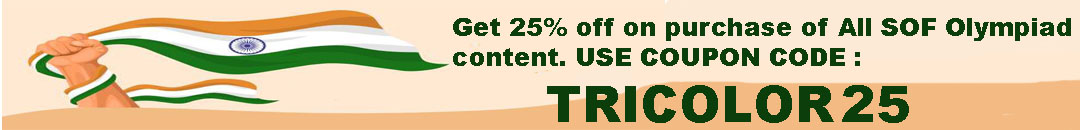# User ForumSubject :IMO    Class : Class 3

## Ans 1:

Class : Class 6
Should be 20 Kg. The mass of 3 balls together is 15 kg implies that weight of one ball is 5 kg. Now dog is equal to 30 kg since there are 6 balls on balance. So deducting mass of two balls which equals (5+5=10 kg), it will be 20 kg.

## Ans 2:

Class : Class 6
30kg

Subject :IMO    Class : Class 3

Class : Class 1
Yes, it is.

Subject :IMO    Class : Class 4

Class : Class 4
But how?

Class : Class 8

## Ans 3:

Class : Class 4
very good beta

Subject :IMO    Class : Class 4

## Ans 1:

Class : Class 3
I guess the answer should be B - 30th. 30th person will be the 5th 6th person and 3rd 10th person.

Class : Class 7

## Ans 3:

Class : Class 4

Subject :IMO    Class : Class 3

## Ans 1:

Class : Class 8
157 +311=468 468 +166=634 so answer is [C]

## Ans 2:

Class : Class 5
C is correct.Not D

## Ans 3:

Class : Class 4

Subject :IMO    Class : Class 4

Class : Class 5

Class : Class 6
Kartik,rs.500

## Ans 3:

Class : Class 6
Answer B, Kritika by Rs 500

Subject :IMO    Class : Class 4

## Ans 1:

Class : Class 4
x = 4, y =6, z = 8, M = 1 therefore answer is 6

## Ans 2:

Class : Class 4
X = 4,y=6,z=8 and m=1 . Therefore the answer is B (6)

## Ans 3:

Class : Class 4
Ans-D

Subject :IMO    Class : Class 6

## Ans 1:

Class : Class 6
it is d only

Subject :IMO    Class : Class 3

Class : Class 5

Class : Class 4

## Ans 3:

Class : Class 6
Answer is C because that is THE BEST for this situation. If we use Kilograms, the weight will come in fractions and hence is not THE BEST answer.

Subject :IMO    Class : Class 3

Class : Class 2